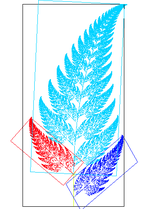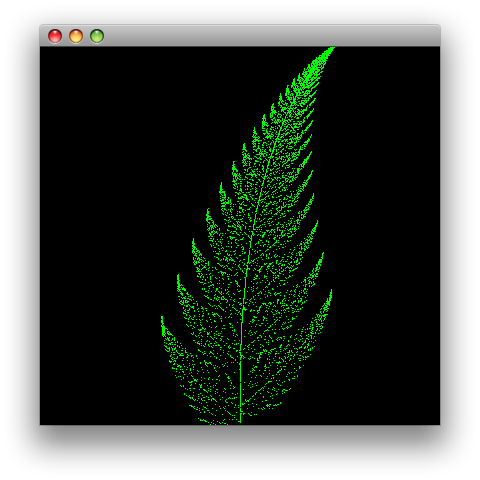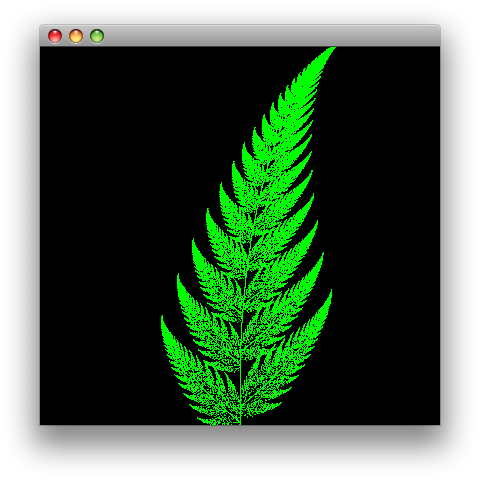## Fractals in Clojure - Fractal Fern

In this post I'm going to show how to draw a type of Fractal called Barnsley's fern.

Basically it goes like this,

• We start at a point, x=0 y=1 in this case
• Paint that point on the canvas.
• Run that point through the transformation function to get the next point.
• Goto step 2 until desired number of points are painted on the canvas.

This example uses the transformation functions from the Wikipedia article on Iterated function systems.

(ns fern
(:import (javax.swing JFrame JLabel)
(java.awt.image BufferedImage)
(java.awt Dimension Color)))

(defn transform-one [[x y]]
[0 (* 0.16 y)])

(defn transform-two [[x y]]
[(- (* 0.2  x) (* 0.26 y))
(+ (* 0.23 x) (* 0.22 y))])

(defn transform-three [[x y]]
[(+ (* -0.15 x) (* 0.28 y))
(+ (* 0.26  x) (* 0.24 y) 0.44)])

(defn transform-four [[x y]]
[(+ (* 0.85   x) (* 0.04 y))
(+ (* -0.004 x) (* 0.85 y) 1.6)])

(defn transform
"Transform point accourding to the percentage."
[target]
(let  [percentage (rand-int 101)]
(cond
(<= percentage 1) (transform-one target)
(<= percentage 7) (transform-two target)
(<= percentage 14) (transform-three target)
(<= percentage 100) (transform-four target))))


We have four transformations, each transformation is selected at random.

• Transformation one is selected 1% of the time.
• Transformation two is selected 7% of the time.
• Transformation three is selected 7% of the time.
• Transformation four is selected 85% of the time.

Transformation one draws the stem. Transformation two draws the bottom frond on the left. Transformation three draws the bottom frond on the right. Transformation four generates successive copies of the stem and bottom fronds to make the complete fern.We can call transform repeatedly to get points to draw on the canvas. We can use loop for this but there is better way.

(defn paint-point
"Paint point on canvas"
[width height [x y] graphics]
(let  [scale (int (/ height 11))
y (- (- height 25) (* scale y))
x (+ (/ width 2)   (* scale x))]
(.drawLine graphics x y x y)))

(defn draw-fern [width height max-points graphics]
(doseq [coord (take max-points (iterate transform [0 1]))]
(paint-point width height coord graphics)))


We use iterate,

user=> (doc iterate)
-------------------------
clojure.core/iterate
([f x])
Returns a lazy sequence of x, (f x), (f (f x)) etc. f must be free
of side-effects


Iterate takes a function and a initial value and returns an infinite sequence of coordinates.

({:x 0, :y 1} {:x 0.28, :y 0.6799999999999999} {:x 0.2652, :y 2.17688} ...


No looping required. It applies the result of each function to the function so that we get a vector of values. Then we take the number of points we want from the sequence.

(defn draw [width height points]
(let [image  (BufferedImage. width height BufferedImage/TYPE_INT_RGB)
canvas (proxy [JLabel] []
(paint [g]
(.drawImage g image 0 0 this)))
graphics (.createGraphics image)]

(.setColor graphics Color/green)
(draw-fern width height points graphics)

(doto (JFrame.)